# ISEE Middle Level Math : How to find the distributive property

## Example Questions

### Example Question #21 : How To Find The Distributive Property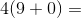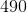Explanation:

First complete the operations within the parentheses: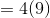Then, multiply: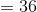Answer:### Example Question #22 : How To Find The Distributive Property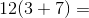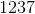Explanation:

First complete the operations in parentheses: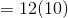Then, multiply: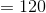Answer:### Example Question #581 : Numbers And Operations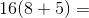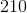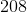Explanation:

First add the numbers in the parentheses. Then multiply: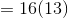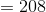Answer:### Example Question #21 : How To Find The Distributive Property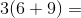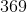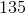Explanation:

Using the distibutive property, solve: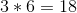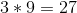Then, solve the equation: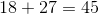Answer:### Example Question #21 : Distributive Property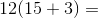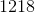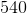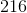Explanation:

Using the distibutive property, solve: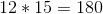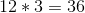Then, solve the equation: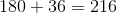Answer:### Example Question #591 : Numbers And Operations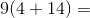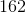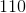Explanation:

Using the distibutive property, solve: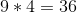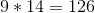Then, solve the equation: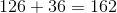Answer:### Example Question #21 : How To Find The Distributive Property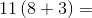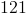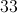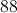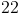Explanation:

First complete the addition in the parentheses, then multiply the two values:

11 (8 + 3)

= 11 (11)

= 121

### Example Question #22 : How To Find The Distributive Property

Solve using the distributive property: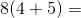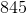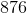Explanation:

First, multiply: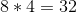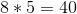Then, add: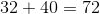Answer:.

### Example Question #23 : Distributive Property

Solve using the distributive property: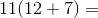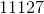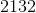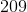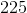Explanation:

First, multiply: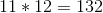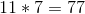Then, add: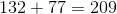Answer:.

### Example Question #22 : How To Find The Distributive Property

Solve the equation.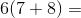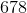Explanation:

Use the distributive property.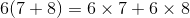Order of operations means that the multiplication must come first.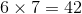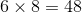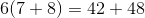Then, solve the equation: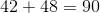Answer:### All ISEE Middle Level Math Resources